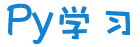注册    登录创作新主题

Python
 python开源   Django   Python   DjangoApp   pycharm
DATA
 docker   Elasticsearch

 问与答   闲聊   招聘   翻译   创业   分享发现   分享创造   求职   区块链   支付之战
aigc
 aigc   chatgpt
WEB开发
 linux   MongoDB   Redis   DATABASE   NGINX   其他Web框架   web工具   zookeeper   tornado   NoSql   Bootstrap   js   peewee   Git   bottle   IE   MQ   Jquery

Python88.com
 反馈   公告   社区推广

Py学习  »  Python

# 真正的Python多线程来了！

CSDN • 6 月前 • 95 次点击【CSDN 编者按】IBM工程师Martin Heinz发文表示，Python即将迎来了真正的多线程时刻！

32岁的Python依然没有真正的并行性/并发性。然而，这种情况即将发生改变，因为在即将发布的Python 3.12中引入了名为"Per-Interpreter GIL"（全局解释器锁）的新特性。在距离发布还有几个月的时间（预计2023年10月发布），但相关代码已经有了，因此，我们可以提前了解如何使用子解释器API编写真正的并发Python代码。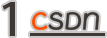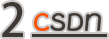````# https://devguide.python.org/getting-started/setup-building/#unix-compiling``git clone https://github.com/python/cpython.git``cd cpython``./configure --enable-optimizations --prefix=\$(pwd)/python-3.12``make -s -j2``./python``# Python 3.12.0a7+ (heads/main:22f3425c3d, May 10 2023, 12:52:07) [GCC 11.3.0] on linux````# Type "help", "copyright", "credits" or "license"

C-API在哪里？

``# Choose one of these:``import _xxsubinterpreters as interpreters``from test.support import interpreters``

``````

from textwrap import dedent````import os``# https://github.com/python/cpython/blob/``#   15665d896bae9c3d8b60bd7210ac1b7dc533b093/Lib/test/test__xxsubinterpreters.py#L75``def _captured_script(script):``    r, w = os.pipe()``    indented = script.replace('\n', '\n                ')``    wrapped = dedent(f"""``        import contextlib``        with open({w}, 'w', encoding="utf-8") as spipe:``            with contextlib.redirect_stdout(spipe):``                {indented}``        """)``    return wrapped, open(r, encoding="utf-8")``def _run_output(interp, request, channels=None):``    script, rpipe = _captured_script(request)``    with rpipe:``        interp.run(script, channels=channels)``        return rpipe.read()````

``from test.support import interpreters``main = interpreters.get_main()``print(f"Main interpreter ID: {main}")``# Main interpreter ID: Interpreter(id=0, isolated=None)``interp = interpreters.create()``print(f"Sub-interpreter: {interp}")``# Sub-interpreter: Interpreter(id=1, isolated=True)``# https://github.com/python/cpython/blob/``#   15665d896bae9c3d8b60bd7210ac1b7dc533b093/Lib/test/test__xxsubinterpreters.py#L236``code = dedent("""``            from test.support import interpreters``            cur = interpreters.get_current()``            print(cur.id)``            """)``out = _run_output(interp, code)``print(f"All Interpreters: {interpreters.list_all()}")``# All Interpreters: [Interpreter(id=0, isolated=None), Interpreter(id=1, isolated=None)]``print(f"Output: {out}")  # Result of 'print(cur.id)'``# Output: 1``

``interp = interpreters.create()``interp.run(code)``

``Fatal Python error: PyInterpreterState_Delete: remaining subinterpreters``Python runtime state: finalizing (tstate=0x000055b5926bf398)``

``def cleanup_interpreters():``    for i in interpreters.list_all():``        if i.id == 0:  # main``            continue``        try:``            print(f"Cleaning up interpreter: {i}")``            i.close()``        except RuntimeError:``            pass  # already destroyed``cleanup_interpreters()``# Cleaning up interpreter: Interpreter(id=1, isolated=None)``# Cleaning up interpreter: Interpreter(id=2, isolated=None)``

``import threading``def run_in_thread():``    t = threading.Thread(target=interpreters.create)``    print(t)``    t.start()``    print(t)``    t.join()``    print(t)``run_in_thread()``run_in_thread()``# ``# ``# ``# ``# ``# ``

````import time````def run_in_thread

():````    interp = interpreters.create(isolated=True)``    t = threading.Thread(target=_run_output, args=(interp, dedent("""``            import _xxsubinterpreters as _interpreters``            cur = _interpreters.get_current()``            import time``            time.sleep(2)``            # Can't print from here, won't bubble-up to main interpreter``            assert isinstance(cur, _interpreters.InterpreterID)``            """)))``    print(f"Created Thread: {t}")``    t.start()``    return t``t1 = run_in_thread()``print(f"First running Thread: {t1}")``t2 = run_in_thread()``print(f"Second running Thread: {t2}")``time.sleep(4)  # Need to sleep to give Threads time to complete``cleanup_interpreters()````

Channels

``# https://github.com/python/cpython/blob/``#   15665d896bae9c3d8b60bd7210ac1b7dc533b093/Lib/test/test_interpreters.py#L583``r, s = interpreters.create_channel()``print(f"Channel: {r}, {s}")``# Channel: RecvChannel(id=0), SendChannel(id=0)``orig = b'spam'``s.send_nowait(orig)``obj = r.recv()``print(f"Received: {obj}")``# Received: b'spam'``cleanup_interpreters()``# Need clean up, otherwise:``# free(): invalid pointer``# Aborted (core dumped)``

``import test.support``def run_in_thread(script):``    test.support.run_in_subinterp_with_config(``        script,``        use_main_obmalloc=True,``        allow_fork=True,``        allow_exec=True,``        allow_threads=True,``        allow_daemon_threads=False,``        check_multi_interp_extensions=False,``        own_gil=True,``    )``code = dedent(f"""``            from test.support import interpreters``            cur = interpreters.get_current()``            print(cur)``            """)``run_in_thread(code)``# Interpreter(id=7, isolated=None)``run_in_thread(code)``# Interpreter(id=8, isolated=None)``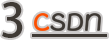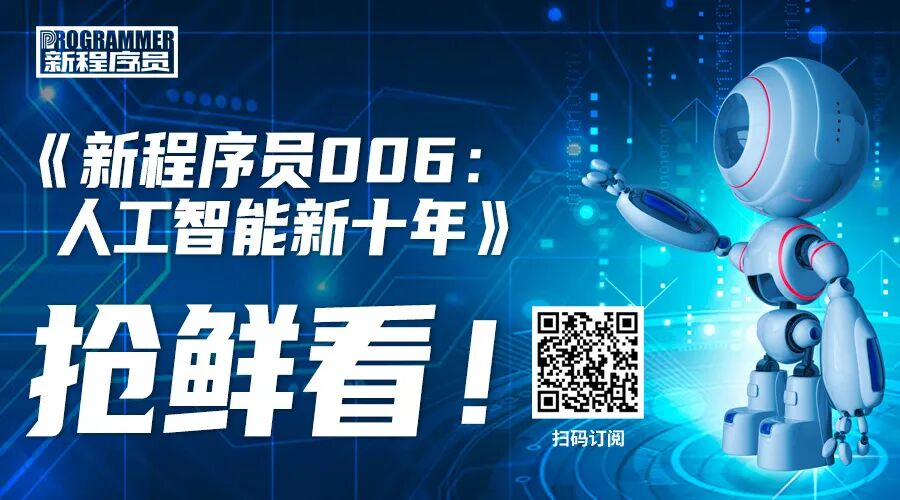Python社区是高质量的Python/Django开发社区